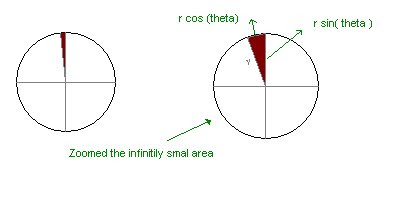## Monday, November 3, 2008

Finding area of Circle.

A few months back i decided to study calculas. I don't want to solve equations. But understanding the concept like infinitily small things and how we can use that in practical purposes. I got a book , which explains very basically the concepts..

As a start of the study i decided to find the area of circle. Everyone knows it .. It is Pi * r ^2.
How it came ? Old people know it . Old indian mathematicians , egyptions all know that.. But calculas is developed after that.. By Sir Newtorn & Sir Lebnitz.

I decided to use caluclas to find the area of circle. We know the cirlcle can be divided in to many triangles see the follwing pictureyou can see that a cirlcle containing a triangle. Suppose if we could divide the circle with very small tiny ! triangle , the area will be equal to that of the sum of all area of the triangles.

Okay this is how Infinitely small items can be useful for practical purpose. Here the area of the one Infinitely small triangle is this ( based on radius r )
Area = 1/2 * r cos(theta) * r sin(theta)
Integrating this to 0-2Pi gives the result , see below for the idea